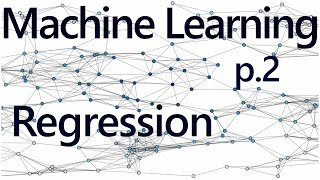# Regression Intro - Practical Machine Learning Tutorial with Python p.2

To begin, what is regression in terms of us using it with machine learning? The goal is to take continuous data, find the equation that best fits the data, and be able forecast out a specific value. With simple linear regression, you are just simply doing this by creating a best fit line.

From h...
To begin, what is regression in terms of us using it with machine learning? The goal is to take continuous data, find the equation that best fits the data, and be able forecast out a specific value. With simple linear regression, you are just simply doing this by creating a best fit line.

From here, we can use the equation of that line to forecast out into the future, where the 'date' is the x-axis, what the price will be.

A popular use with regression is to predict stock prices. This is done because we are considering the fluidity of price over time, and attempting to forecast the next fluid price in the future using a continuous dataset.

Regression is a form of supervised machine learning, which is where the scientist teaches the machine by showing it features and then showing it was the correct answer is, over and over, to teach the machine. Once the machine is taught, the scientist will usually "test" the machine on some unseen data, where the scientist still knows what the correct answer is, but the machine doesn't. The machine's answers are compared to the known answers, and the machine's accuracy can be measured. If the accuracy is high enough, the scientist may consider actually employing the algorithm in the real world.

https://pythonprogramming.net

#machine learning #python #tutorial #artificial intelligence #scikit-learn #theano #tensorflow #regression #linear regression #linear regression code #supervised machine learning## sentdex

### 【予測】200万人まであと2520日（2029年8月31日）

Python Programming tutorials, going further than just the basics. Learn about machine learning, finance, data analysis, robotics, web development, game development and more.

I have ~1000 videos, all of which do not fit on the front page. Try searching on the channel page, or via https://pythonpr...
Python Programming tutorials, going further than just the basics. Learn about machine learning, finance, data analysis, robotics, web development, game development and more.

I have ~1000 videos, all of which do not fit on the front page. Try searching on the channel page, or via https://pythonprogramming.net. If you cannot find something, just ask!

Bitcoin donations: 1GV7srgR4NJx4vrk7avCmmVQQrqmv87ty6
Paypal donations: [email protected]